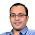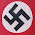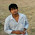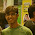### Value of Pi - Estimation using Dice

Source: Asked to a friend at Goldman Sachs Quant Interview

Problem:
Estimate the value of pi using a dice

Update: (21 June 2014)
Solution posted by Tushar Makkar, 'My first amateur attempt', Satadip, Gaurav Bajaj and me (Pratik) in comments. Thanks

1.Imagine we have a dice and we have to throw in a circle embedded in a 4*4 square. This circle touches the boundaries of this square. Let’s say out if N trials number of times this dice falls on circle is C and number of times this dice falls on square is N.
So the ratio of C/N will represent the ratio of area of circle to the area of square which is [PI*(R*R) ] /(4*4).
(C/N)=(PI*4/16)
[ C/N=PI/4 ] => PI=4*C/N
At this point, we will perform the simulation using N=3000. So, the trick is number of times the dice falls on square is equal to number of trials performed. The range of x and y will vary from -2 and 2.
And the condition which is used in simulation to check whether the dice falls inside the circle or not is IF(sqrt(x^2+y^2) <2) then assign cell’s value is 1 and if the condition fails, assign cell’s value as 0 . In the end we can use find out numbers of cells containing 1 to find C/N ratio.
Using both the equations we can find approximate value of PI . These kind of problem solving methods are known as Monte Carlo simulation techniques .

1.Correct Solution. Thanks

2.consider a circle inscribed in a square , the probability of a random point from the square also in the circle is pi/4
Now how do we actually pick up a point randomly , this is where the dice will help
break the square into 6 by 6 blocks , 2 rolls of dice will give us co-ordinate of the box
now if we stop the experiment here give the center of the square as the random point else apply the same experiment to this smaller box .
choosing any of the 36 smaller boxes is equally likely so if we do enough trials we can be sure to pick a random point with every point being equally likely.
This solution was by my friend Sankeerth(EE , IITB class of 2009)

1.Correct Solution. Thanks

3.The die is meant to mislead you. You can simply throw any object randomly in a square and estimate the probability that it lands inside an embedded circle. If the square is of side 2 and the circle of radius 1, then the probability is pi/4.

1.Gowtham, Nope. Its not a trick question. Please check the answer. Interesting aha moment. :-)

2.How is this method specific to a dice. Any other object would suffice, No?

4.use monte carlo simulations.... take a square [-3, 3] x [-3, 3] and check a pair of die-rolls(dey give the co-ordinates of point) a large no of times.

1.Yep. Correct. Thanks

5.Here's a little bit neat method.
Throw a die six times. Let the values be a, b, c, d, e, f.
Define a event X if {(a-1)/6 + (b-1)/36 + (c-1)/216}^2 + {(d-1)/6 + (e-1)/36 + (f-1)/216}^2 <1 and event Y is the otherwise.
Calculate {the number of event X} / {(the number of X)+(the number of Y)}
This will converges approximately pi.

1.2.6.A more accurate solution:
divide the square in 6x6 blocks and each square again into 6x6 sub-squares as shown in the image below
http://postimg.org/image/xiizzkp2p/
Throw the dice:
1. if the dice points to the square such that it lies in the centre 4x4 square add one to Pi_count variable
2. if the dice points to the 20 squares on the perimeter, throw dice one more time and if the dice points to subsquare which is filled with blue add one to Pi_count.

Value of Pi = Pi_count/Num_of_iteration*4

The precision improved 6 times with 1.55 times increase in no of dice throws.

1.Yep. Correct. Thanks

7.Generate two such random numbers, x and y, appending digits to each until there are enough digits in both numbers to establish with certainty whether x^2+y^2<1 or x^2+y^2>1. (Equality occurs with probability 0 and can be disregarded.) If x^2+y^2<1, add a tally to the "in" column; otherwise add a tally to the "out" column.

After generating a n=in+out such pairs, and so accumulating a total of n tallies, we have
π ~ 4*in/(in+out).
The idea here is that x and y determine a random point in the square [0,1]^2 that is uniformly distributed. The area of the quarter-circular region x^2+y^2<1 is π/4, and so a uniformly selected point in the square will lie in that region with probability π/4.

1.sir do we need to know algorithmic techniques before solving your puzzles....i have jst passed out class 12th

8.Suppose you have 3 die (A, B, C):

Calculate expected value of: {the actual number on die A or 1} (choose 1 if sum of numbers on B + C = 5)